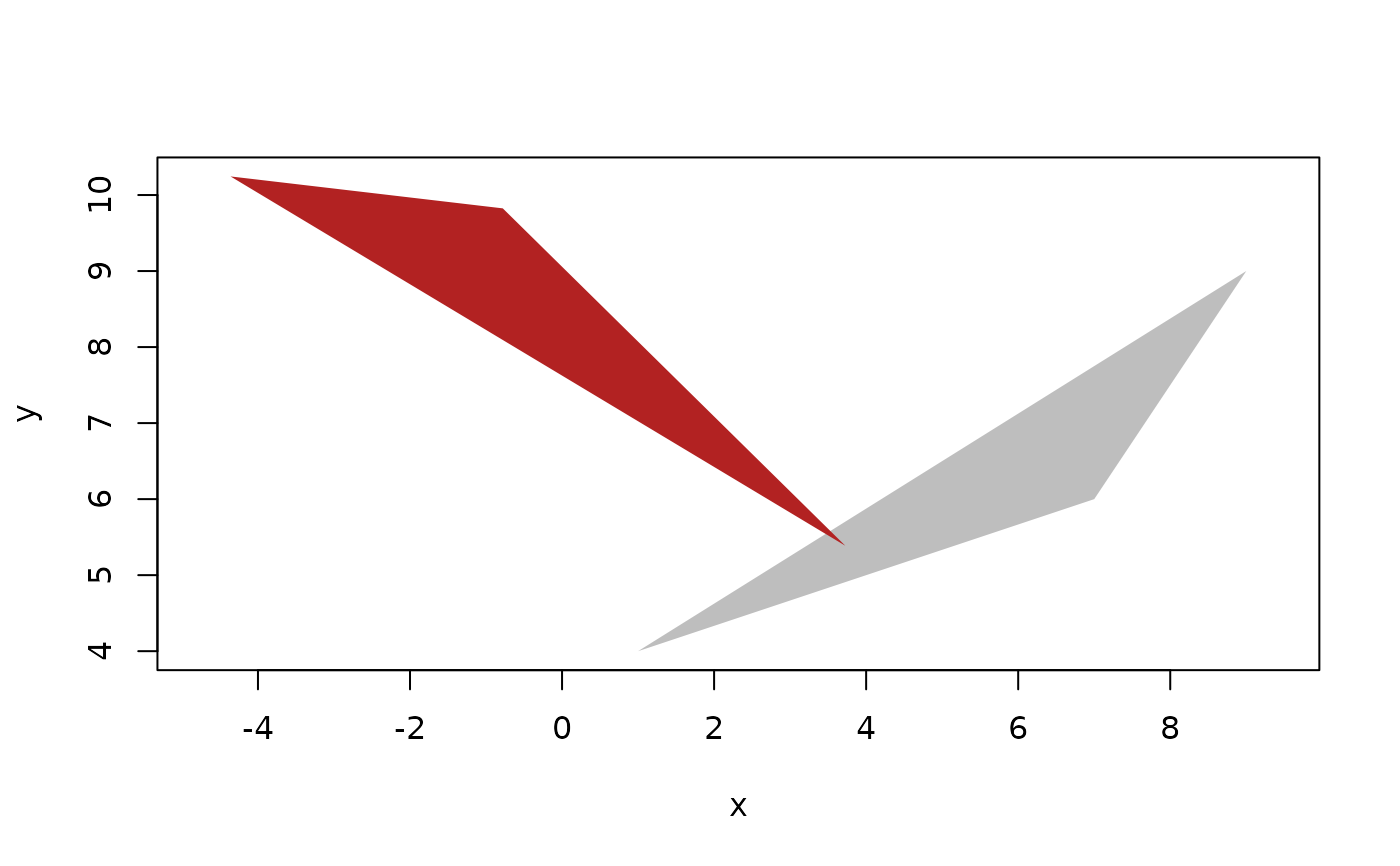These functions allow you to create vectors of transformation matrices for affine transformation in 2 or 3 dimensions. Transformation matrices are used to apply transformations to geometries using transform(). Transformations can be stacked by multiplying them together. This is generally more performant than applying transformations one by one to geometries. Transformations can be reversed by applying the inverse transformation to a geometry. The inverse transformation matrix can be obtained using inverse(). Affine transformation matrices have an additional column and row compared to linear transformation matrices. This means that matrices for 2 dimensional transformations are 3x3 and matrices for 2 dimensional transformations are 4x4. In both cases the last row will consist of 0's and a final scaling factor (usually 1). Rotation is generally not possible to do while maintaining exactness as sine and cosine cannot be calculate to exactness. 3 dimensional rotation can either be done around an axis, around a direction, or be defining specific angles of rotation for yaw, pitch, and roll.

## Usage

affine_identity(dim = 2L)

affine_matrix(mat)

affine_translate(vec)

affine_scale(fac, dim = 2L)

affine_scale2(x = 1, y = 1, z = NA, dim = NA)

affine_shear(
x = NA,
y = NA,
xy = NA,
xz = NA,
yx = NA,
yz = NA,
zx = NA,
zy = NA
)

affine_reflect(x = FALSE, y = FALSE, z = FALSE, dim = 2L)

affine_rotate(rho, axis = NA, direction = NA, yaw = NA, pitch = NA, roll = NA)

is_affine_transformation(x)

as_affine_transformation(x)

inverse(x)

is_reflecting(x)

## Arguments

dim

The dimensionality of the transformation matrix

mat

An object that can be converted to an affine transformation matrix vector. Matrices and arrays can be converted provided they have the correct dimensions. List of matrices can be converted provided that all matrices have the same dimensions and that the dimensions is correct

vec

A vector of vectors or an object convertible to one

fac

A scaling factor to apply

x, y, z, xy, xz, yx, yz, zx, zy

Scaling and shearing factors for each separate dimension/plane, or flags to indicate whether to reflect on the given axis

rho

An angle in radians to rotate (counterclockwise)

axis

For 3 dimensional rotation, which axis to rotate around

direction

A direction vector or an object convertible to one

yaw, pitch, roll

Angles in radians for yaw, pitch, and roll rotation.

## Value

An euclid_affine_transformation vector

## Note

Circles and spheres only transforms correctly with euclidean transformations (i.e. translation, rotations, and reflection) as well as scaling by the same factor along all dimensions (as provided by affine_scaling()). Shearing and stretching/squeezing will only affect the location of the center, not the circularity of the geometry.

Other non-geometry vectors: bbox(), exact_numeric()

## Examples

# Rotate triangle around its centroid and then around the center
p <- point(sample(10, 3), sample(10, 3))
t <- triangle(p, p, p)

ct <- centroid(t)
# Assemble transformation (remember reverse order)
trans <- affine_rotate(pi/4) *
affine_translate(vec(ct)) *
affine_rotate(2*pi/5) *
affine_translate(-vec(ct))

t2 <- transform(t, trans)

plot(c(t, t2), col = c("grey", "firebrick"), border = NA)